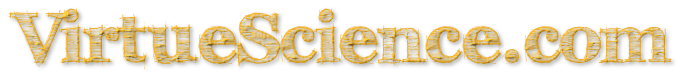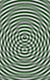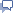<230 Number Data-BaseRandom Number232>

## The Number 231 : Properties and Meanings

Prime Factors of 231 =3x7x11.

231 is a Triangular Number.

231 is a Hexagonal Number.

231 is an Octahedral Number.

231 is a 17-gonal Number.

231 is a 78-gonal Number.

231 is a Centered 23-gonal Number.

231 is the 22nd number which is the Product of 3 distinct primes.

231 is the triangle of 21 which is the triangle of 6 which is the triangle of 3 which is the triangle of 2. Thus, 231 , is a triangle of a triangle of a triangle.

16 can be Partitioned in 231 ways.

231 =7x33

The 231 two-letter combinations of the 22 letters of the Hebrew Alphabet refered to as the 231 gates of Sefer Yetzirah.

Two hundred thirty-one is the exact number of cubic inches in a U.S. liquid gallon.

 <230 Number Data-BaseRandom Number232>Share any properties and meanings for particular Numbers...contact me directly, thanks.